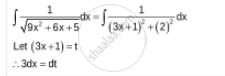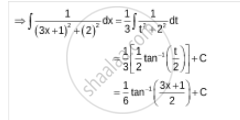Share

# Integrate the Functions 1/Sqrt(9x^2 + 6x + 5) - CBSE (Commerce) Class 12 - Mathematics

ConceptIntegrals of Some Particular Functions

#### Question

Integrate the functions 1/sqrt(9x^2 + 6x + 5)

#### SolutionIs there an error in this question or solution?

#### Video TutorialsVIEW ALL 

Solution Integrate the Functions 1/Sqrt(9x^2 + 6x + 5) Concept: Integrals of Some Particular Functions.
S